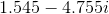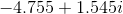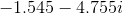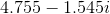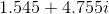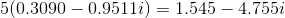# Precalculus : Express Complex Numbers In Rectangular Form

## Example Questions

### Example Question #1 : Express Complex Numbers In Rectangular Form

Convert the following to rectangular form: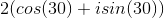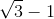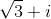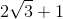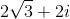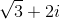Explanation:

Distribute the coefficient 2, and evaluate each term: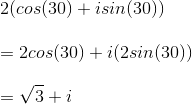### Example Question #2 : Express Complex Numbers In Rectangular Form

Convert the following to rectangular form: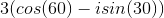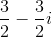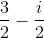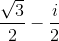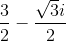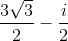Explanation:

Distribute the coefficient and simplify: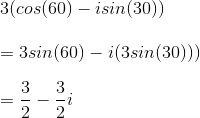### Example Question #3 : Express Complex Numbers In Rectangular Form

Represent the polar equation: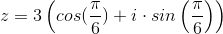in rectangular form.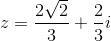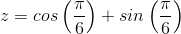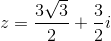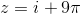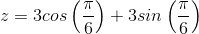Explanation:

Using the general form of a polar equation: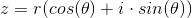we find that the value ofisand the value of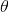is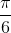.

The rectangular form of the equation appears as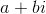, and can be found by finding the trigonometric values of the cosine and sine equations.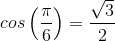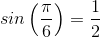distributing the 3, we obtain the final answer of: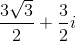### Example Question #4 : Express Complex Numbers In Rectangular Form

Represent the polar equation: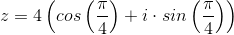in rectangular form.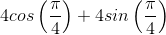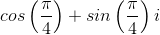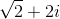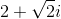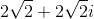Explanation:

Using the general form of a polar equation:we find that the value of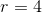and the value of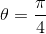. The rectangular form of the equation appears as, and can be found by finding the trigonometric values of the cosine and sine equations.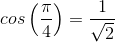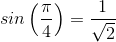Distributing the 4, we obtain the final answer of: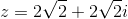### Example Question #5 : Express Complex Numbers In Rectangular Form

Represent the polar equation: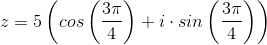in rectangular form.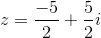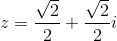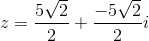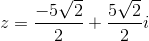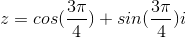Explanation:

Using the general form of a polar equation: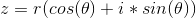we find that the value of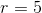and the value of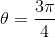. The rectangular form of the equation appears as, and can be found by finding the trigonometric values of the cosine and sine equations.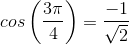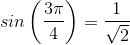distributing the 5, we obtain the final answer of: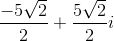### Example Question #6 : Express Complex Numbers In Rectangular Form

Convert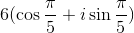in rectangular form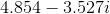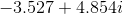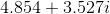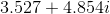Explanation:

To convert, just evaluate the trig ratios and then distribute the radius.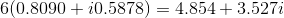### Example Question #7 : Express Complex Numbers In Rectangular Form

Convert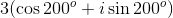to rectangular form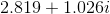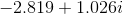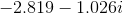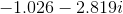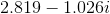Explanation:

To convert to rectangular form, just evaluate the trig functions and then distribute the radius: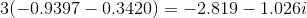### Example Question #8 : Express Complex Numbers In Rectangular Form

Convert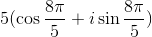to rectangular form